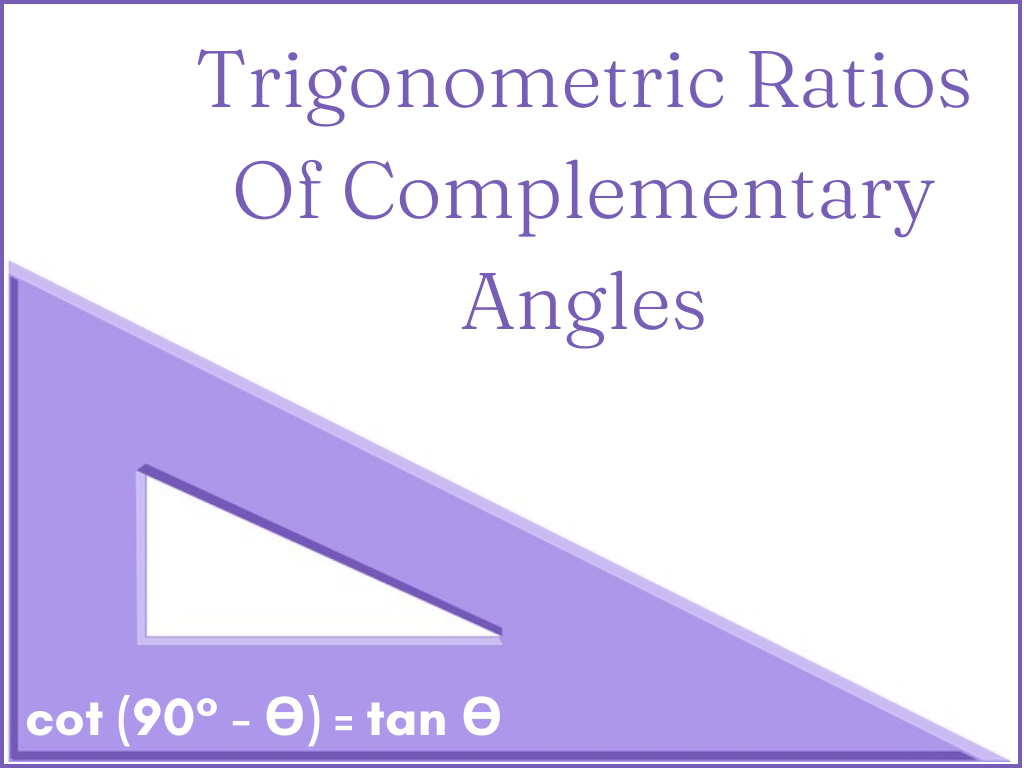## Trigonometric Ratios of Complementary Angles Class 10th

Introduction When the sum of two angles is 90° they are called Complementary Angles. If any acute angle is A then its complementary angle will be (90° – A). In a Right-angled triangle, Trigonometric Ratios of Complementary Angles are found as follows. Explanation Let △PQR be a right-angled triangle in which ∠Q is the right …